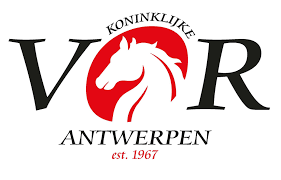OFFICIAL RESULTS LIST## 07 95cm klasse 3 Barema A - 2 fazen speciaal - 274.2.5 Geldt voor Lannoo Cup

Result list: 53 competitors in this competition. Last updated at 11:29 hrs (30.03.2019)

Rank
Nation
Competitor
CNR
Horse
 Phase 1Fehler Phase 2Fehler TotalFehler Zeit
1
 0 0 = 0.00 29.26
2
 0 0 = 0.00 30.78
3
YARI VAN DEN BRANDEN,
 0 0 = 0.00 31.93
4
 0 0 = 0.00 32.91
5
 0 0 = 0.00 33.41
6
 0 0 = 0.00 34.7
7
 0 0 = 0.00 37.39
8
 0 0 = 0.00 38.7
9
 0 0 = 0.00 38.77
10
 0 0 = 0.00 40.87
11
 0 0 = 0.00 44.24
12
 0 1 = 1.00 45.98
13
 0 1 = 1.00 46.2
14
 0 1 = 1.00 46.45
15
 0 1 = 1.00 47.38
16
 1 1 = 2.00 45.94
17
 0 2 = 2.00 51.56
18
 0 4 = 4.00 28.97
19
 0 4 = 4.00 32.58
20
 0 4 = 4.00 32.99
21
 4 0 = 4.00 34.96
22
 0 4 = 4.00 36.72
23
 0 4 = 4.00 37.21
24
 4 0 = 4.00 38.56
25
 0 4 = 4.00 39.03
26
 0 4 = 4.00 40.17
27
 4 0 = 4.00 41.16
28
 0 4 = 4.00 41.48
29
 4 0 = 4.00 42.21
30
 4 0 = 4.00 44.09
31
 4 1 = 5.00 47.45
32
 4 2 = 6.00 52.94
33
 4 4 = 8.00 37.26
34
 4 4 = 8.00 43.03
35
 0 9 = 9.00 45.42
36
 4 5 = 9.00 47.22
37
 4 5 = 9.00 47.24
38
 4 5 = 9.00 48.22
39
 0 9 = 9.00 62.74
40
SILKE VAN LANGENHOVEN,
 0 9 = 9.00 63.63
41
 4 7 = 11.00 54.36
42
 8 4 = 12.00 40.31
43
 7 5 = 12.00 45.81
44
 1 12 = 13.00 40.75
45
 14 5 = 19.00 47.45
 0 0 eliminated
 0 4 eliminated
 4 4 eliminated
 4 12 retired
 0 eliminated
 0 4 eliminated
 4 eliminated
 4 eliminated